Click in-app to access the full platform documentation for your version of DataRobot.

# Coefficients (preprocessing)¶

The Coefficients tab provides a visual indicator of information that can help you refine and optimize your models. If you are using a regression model, use the Coefficients tab provides a visual representation of the 30 most important variables, sorted (by default) in descending order of impact on the final prediction. Variables with a positive effect are displayed in red; variables with a negative effect are shown in blue. The tab also provides, for supported models, a link that allows you to export the parameters and coefficients DataRobot uses with the selected model to generate predictions. (Exported coefficients includes all coefficients, not just the top 30.)

Note that the Coefficients tab is only available for a limited number of models because it is not always possible to derive the coefficients for complex models in short analytical form.

The Coefficients chart determines the following to help assess model results:

• Which features were chosen to form the prediction in the particular model?
• How important is each of these features?
• Which features have positive and negative impact?

Tip

The Leaderboard > Coefficients and Insights > Variable Effects charts display the same type of information. Use the Coefficients tab to display coefficient information while investigating an individual model; use the Variable Effects chart to access, and compare, coefficient information for all applicable models in the project.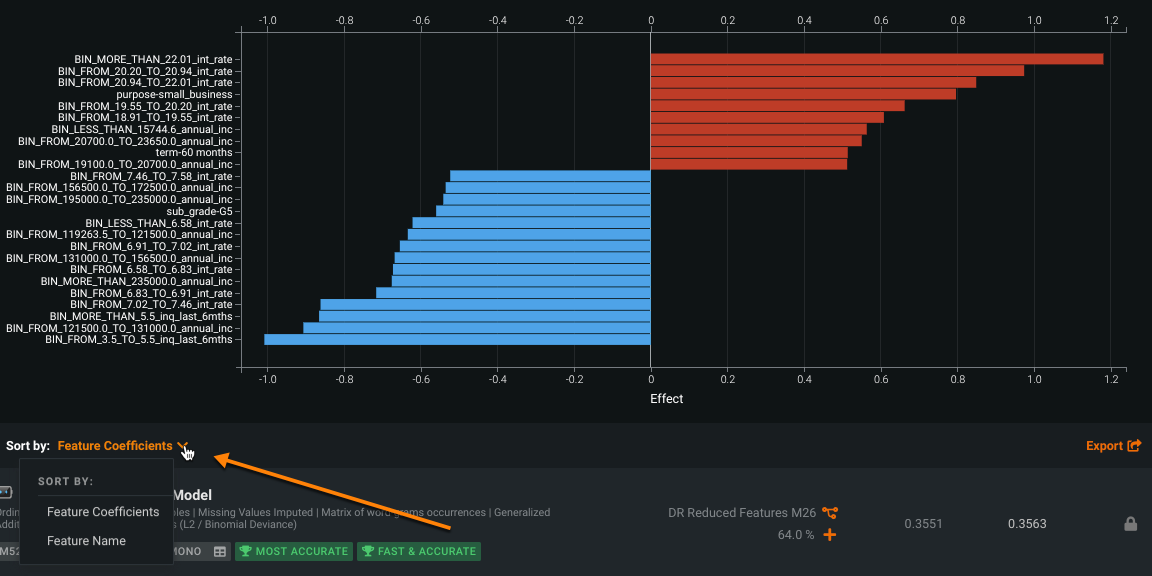Time series projects have an additional option to filter the display based on forecast distance, as described below.

See below for more detailed ways to consider Coefficients chart output.

## Use the Coefficient chart¶

The Coefficients chart opens when you click the Coefficients tab. Actions available include:

1. Click the Sort By dropdown to set the sort criteria, either Feature Coefficients or Feature Name:

• Feature Coefficients: Sorts in descending order of impact on the final prediction.
• Feature Name: Sorts features alphabetically.
2. Click the Export button to access a pop-up that allows download of a chart PNG, a CSV file containing feature coefficients, or both in a ZIP file.

Tip

If a model has the ability to produce rating tables (for example, GAM and GA2M), the CSV download option is not available. Use the Rating Tables tab instead. (These models are indicated with the rating table icon on the Leaderboard.)

3. If the main model uses a two-stage modeling process (Frequency-Severity Elastic Net, for example), you can use the dropdown to select a stage. DataRobot then graphs parameters corresponding to the selected stage.

## Preprocessing and parameter view¶

Exporting coefficients with preprocessing information provides the data needed to reproduce predictions for a selected model. With the click of a link, DataRobot generates a table of model parameters (coefficients and the values of the applied feature transformations) for the input data of supported models. That is, while you can export coefficients for all models showing the Coefficients tab, not all models showing the tab allow you to export preprocessing information. DataRobot then builds a CSV table of a model's parameter, transformation, and coefficient information. There are many reasons for using coefficients with preprocessing information, for example, to replicate results manually for verification of the DataRobot model.

### Generate output¶

DataRobot automatically generates coefficients with preprocessing information, and makes it available for export, for all supported models when you use any of the supported modeling modes.

Tip

Generalized Additive Models (GA2M) using pairwise interactions, typically used by the insurance industry, generate a different rating table for export. For more information, see the sections on exporting and/or interpreting export output for GA2M.

To use the feature:

1. Use the Leaderboard search feature to list all models with coefficient/preprocessing information available by searching the term "bi".
2. Select and expand the model for which you want to view model parameters.
3. Click the Coefficients tab to see a visual representation of the thirty most important variables. Click the Export button and select .csv from the available export options.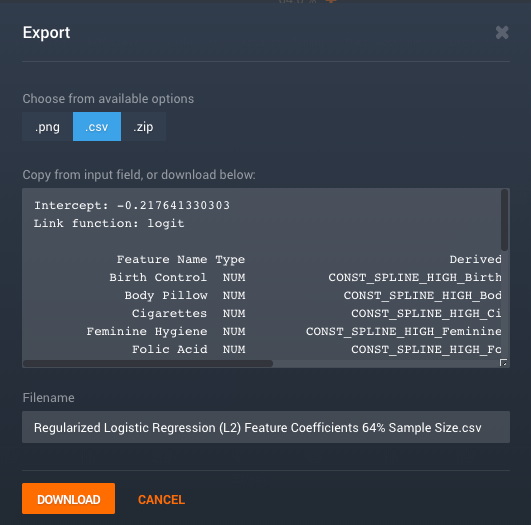4. Inspect the parameter information displayed in the box. To save the contents in CSV format, click the Download button and select a location.

5. If your data contains text features, either all text or in combination with numerical and/or categorical features, continue to the section on using coefficient/preprocessing information with text variables.

See the information below for a detailed description of the export output and how to interpret it.

The following sections provide information on:

## Chart display based on forecast distance¶

Because DataRobot creates so many additional features when building a time series modeling dataset with multiple forecasting distances, displaying parameters for all forecast distances at once would result in a difficult viewing experience. To simplify and make the view more meaningful, use the Forecast distance selector to view coefficients for a single distance. Set the distance by either clicking the down arrow to expand a dialog or clicking through the distance options with the right and left arrows.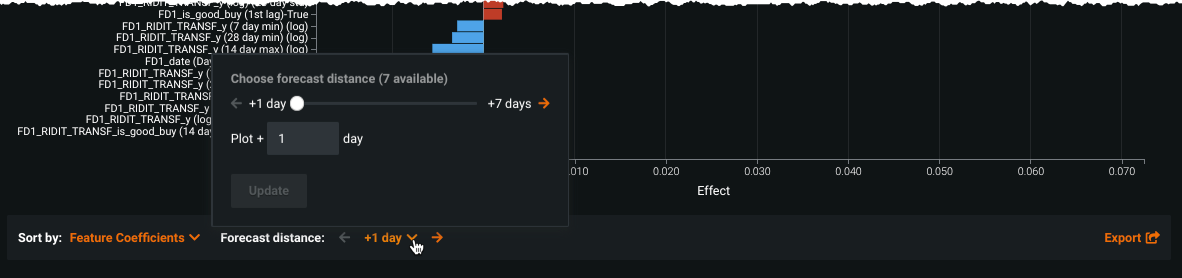Additionally, with multiseries modeling where Performance Clustered and Similarity Clustered models were built, the chart displays cluster information that includes the number of series found in each cluster (up to 20 clusters). This information can be described from the coefficients and transparent parameters and support producing user-coded insights outside of DataRobot. For example, with this information you could reproduce most of the results with an XGB model by making a new dataset that includes only series from specific clusters. For other non-cluster multiseries models, the display is the same as described above.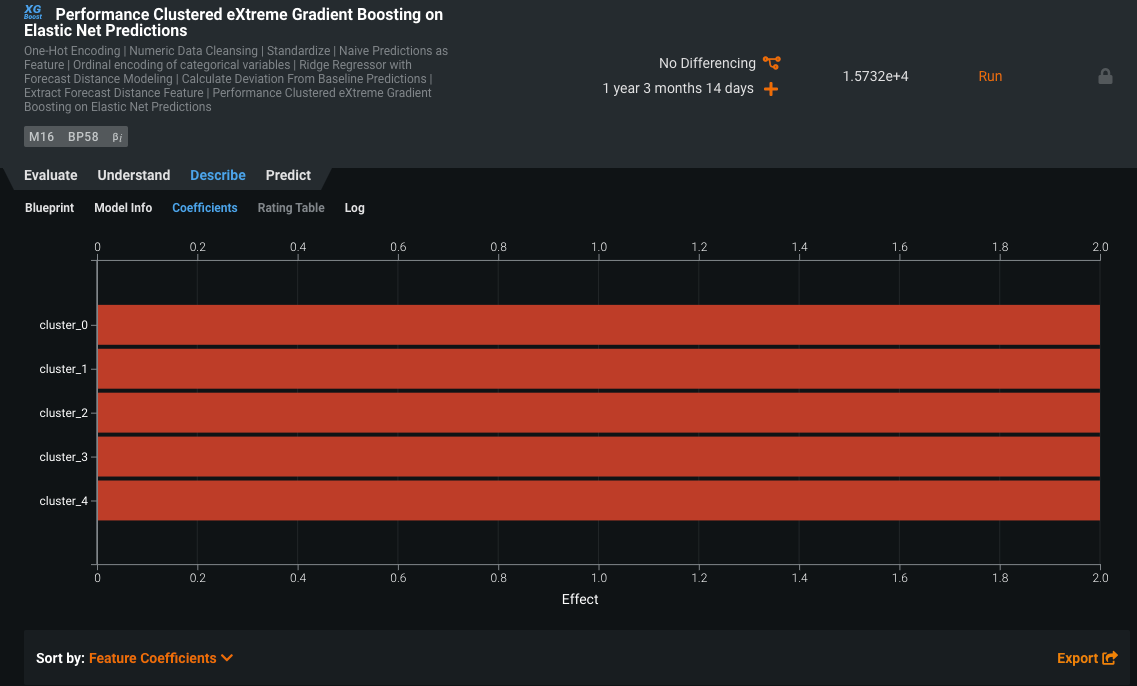To support datasets with a large number of series, where displaying per-cluster information in the UI would be visually overwhelming, use the export to CSV option. The resulting export will provide a complete mapping of all series IDs to the associated cluster.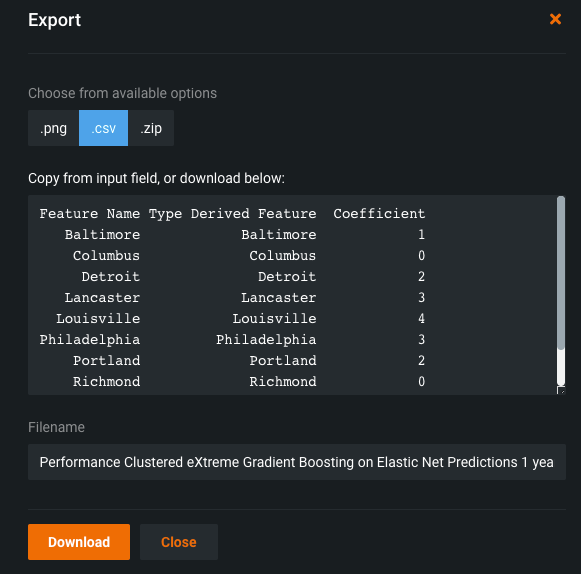## Understand the Coefficient chart¶

With the Coefficients chart open and sorted by rank, consider the following:

1. Look carefully at features that have a very strong influence on your model to ensure that they are not dependent upon the response. Consider excluding these features from the model to avoid target leakage.

2. Try to determine if a particular feature is included in only one of the dozens of models generated by DataRobot. If so, it may not be particularly important. Excluding it from the feature set might help optimize model-building and future predictions.

3. Examine, in both the dataset and the models, any features that have a strongly positive effect in one model and a strongly negative effect in another.

4. Reduce the number of features considered by a model, as it may change the relative importance of each remaining feature. You may find it useful to compare how the importance of each feature changes when a feature list is reduced.

## Why export?¶

You may want—or be required—to view and export the coefficients DataRobot uses to generate predictions. This is an appropriate feature if you need to:

• observe regulatory constraints.

• roll out a prediction solution without using DataRobot. This might be the case in environments where DataRobot is prohibited or not possible, for example in offline deployments such as banks or video games.

• adjust coefficients to control model build.

• quickly verify parameters accuracy without the need to compute it by hand and inspect transformations process.

Example use case: greater model insights

Coefficient/preprocessing information can help with modeling mortality rates for breast cancer survivors. From the parameters perhaps you can come to understand:

• which age ranges are grouped together as similar risks.
• which tumor sizes are grouped together as similar risks, and at exactly what point the risk suddenly increases.

Example use case: regulatory disclosure

A Korean regulator requires all model coefficients and data preprocessing steps used by banks. With DataRobot, the bank can send the coefficient output.

To reproduce the steps DataRobot takes (and illustrates in the model blueprint) to build a model, you must know the formulas used. The export available through the Coefficients tab provides the coefficients and transformation descriptions that paint a picture of how a model works.

Example use case: text-based insights

DataRobot can also work with datasets containing text columns, allowing you to download certain text preprocessing parameters. You may want to use this feature, for example, to align a marketing campaign message with the direct marketing customers selected by your DataRobot model. Using text preprocessing, you can investigate the derived features used in the modeling process to gain an intuitive understanding of selected clients.

### Supported model types¶

The coefficient/preprocessing export feature supports DataRobot's linear models, which are easy to describe in simple, portable tables of parameters. Such parameter tables might allow you to see, for example, that age is the most important variable for predicting a certain event. More complex, non-linear models can be inspected using DataRobot's other built in tools, available from the Feature Impact, Feature Effects, and Prediction Explanations tabs.

DataRobot provides the export feature for regularized and non-regularized GLMs, specifically:

• Generalized Linear Model
• Elastic Net Classifier
• Elastic Net Regressor
• Regularized Logistic Regression

DataRobot supports the following transformations (described in detail below):

• Numeric imputation
• Constant splines
• Polynomial and log transforms
• Standardize
• One-hot encoding
• Binning
• Matrix of token occurrences

In general, more complicated proprietary preprocessing techniques are not exportable. For example, an imputation is exportable, but a polynomial spline is not. In the example below, although both are the same model type, the second model uses Regularized Linear Model Processing, which, because of the preprocessing, is not exportable.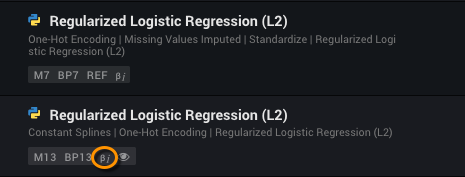DataRobot supports equation exports for Eureqa models, but does not currently support coefficient exports.

### Interpret export output¶

The following is a sample excerpt from coefficient/preprocessing output:

``````1 Intercept: 5.13039673557
2 Loss distribution: Tweedie Deviance
4
5 Feature Name Type Derived Feature          Transform1  Value1   Transform2                     Value2  Coefficient
6           a  NUM  STANDARDIZED_a  Missing imputation 59.5000  Standardize  (56.078125,31.3878483092)       0.3347
7           b  NUM  STANDARDIZED_b  Missing imputation 24.0000  Standardize   (24.71875,15.9133088463)       0.2421
``````

In the example, the Intercept, Loss distribution, and Link function parameters describe the model in general and not any particular feature. Each row in the table describes a feature and the transformations DataRobot applies to it. For example, you can read the sample as follows:

1. Take the feature named "a" (line #6) and replace missing values with the number 59.5.
2. Apply the STANDARDIZED transform formula—the mean (56.078125) and standard deviation (31.3878483092) to the value.
3. Write the result, now a derived feature, to the column "STANDARDIZE_a".
4. Follow the same procedure for feature "b".

The resulting prediction from the model is then calculated with the following formula, where the `inverse_link_function` is the exponential (the inverse of log)and standardized `_a` and `_b` are each multiplied their coefficient (the model output) and then added to the intercept value:

``````resulting prediction = inverse_link_function( (STANDARDIZE_a * 0.3347) + (STANDARDIZE_b * 0.2421) + 5.13)
``````

If the main model uses a two-stage modeling process (Frequency-Severity Elastic Net, for example), two additional columns—`Frequency_Coefficient` and `Severity_Coefficient`—provide the coefficients of each stage.

### Coefficient/preprocessing information with text variables¶

Text-preprocessing transforms text found in a dataset into a form that can be used by a DataRobot model. Specifically, DataRobot uses the Matrix of token occurrences (also known as "bag of words" or "document-term matrix") transformation.

Deepdive: Word Cloud coefficient values

The coefficient value displayed is a rescaling of the linear model coefficients. That is, DataRobot models a row and then changes all its ngrams to be consistent with `minimum in the negative box = -1` and `maximum in the positive box = 1` coefficients. The coefficient value is then a percentage of those observations.

When generating coefficient/preprocessing output, DataRobot simply exports the text preprocessing parameters along with the other parameters.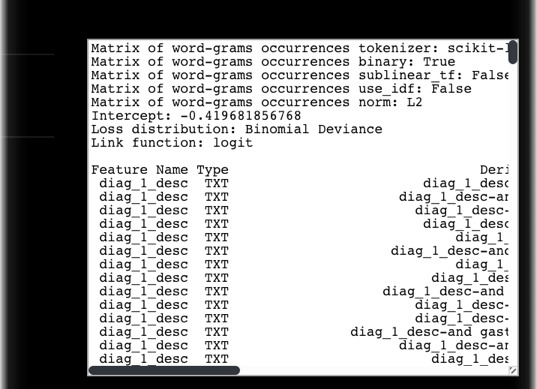When text preprocessing occurs, DataRobot reports the parameters it used in the header section, prefixed with the transform name. You will need these "instructions" to create dataset columns from new text rows. Possible values of the transform name (with and without inverse document frequency (IDF) weighting) are:

• Matrix of word-grams occurrences [with tfidf]
• Matrix of word-grams counts [with tfidf]
• Matrix of char-grams occurrences [with tfidf]
• Matrix of char-grams counts [with tfidf]

The following table describes the parameters (key-value fields) that DataRobot used to create the parameter export. These values are reported at the top of the file:

Parameter name Value Description
tokenizer name Specifies the external library used to perform the tokenization step (e.g., scikit-learn based tokenizer).
binary True or False If True, converts the term frequency to binary value. If False, no conversion occurs.
sublinear_tf True or False If True, applies a transformation 1 + log(tf) to term frequency . If False, does not modify term frequency count.
use_idf True or False If True, applies IDF weighting to the term. If False, there is no change to the weighting factor.
norm L1, L2, or None If L1 or L2, applies row-wise normalization using the L1 or L2 norm.

Each row in the parameters table represents a token. To generate predictions on new data using the coefficients listed in the parameters table, you must first create a document-term matrix (a matrix is the extracted features).

To create features from text:

1. Count the number of occurrences (i.e., term frequencies, <tf>) of each token in the new dataset row. If binary is True, the value is 0 (not present) or 1 (present) for each token. If binary is False, occurrences is the actual token count.

2. If `sublinear_tf` is true, apply the transformation `1 + log(tf)` to the token count.

3. If `use_idf` is true, apply IDF weighting to the token. You can find the IDF weight for the transformation in the Value field of the export. For example, in the tuple (cardiac, 0.01), use the multiplier 0.01.

4. If normalization was used, normalize the resulting feature vector using the appropriate norm.

Once you have extracted the text features for your dataset, you can generate predictions using the coefficients of the linear model.

### Details of preprocessing¶

The following sections describe the routines DataRobot uses to reproduce predictions from the parameters table.

#### Numeric imputation¶

Missing imputation imputes missing values on numeric variables with the number (value).

Value: number Value example: 3.1415926

#### Standardize¶

Standardize standardizes features by removing the mean and scaling to unit variance:

``````x' = (x - mean) / scale
``````

Value: (mean, scale) Value example: (0.124072727273, 0.733724343942)

#### Constant splines¶

Constant splines converts numeric features into piece-wise constant spline base expansion. A derived feature will equal to 1.0 if the original value `x` is within the interval:

``````a < x <= b
``````

Additionally, N/A in original feature will set 1.0 in the derived feature if `value` ends with "(default for NA)" marker.

Value: (a, b] Value examples: (-inf, 8.5], (8.5, 12.5], (12.5, inf)

#### Polynomial and log transforms¶

Best transform applies the formula to the original feature.

If formula contains `log`, negatives are replaced with the median of the remaining positives.

Value: formula operating on the original feature. Value examples: log(a)^2, foo^3

Note

If your target is log transformed, or if the model uses the log link (Gamma, Poisson, or Tweedie Regression, for example), the coefficients are on the log scale, not the linear scale.

#### One-hot encoding¶

One-hot (or dummy-variable) transformation of categorical features.

• If `value` is a string, derived feature will contain 1.0 whenever the original feature equals value.

• If `value` is "Missing value," derived feature will contain 1.0 when the original feature is N/A.

• If `value` is "Other categories," derived feature will contain 1.0 when the original feature doesn't match any of the above.

Value: string, or `Missing value`, or `Other categories` Value example: 'MA', Missing value

#### Binning¶

Binning transforms numerical variables into non-uniform bins.

The boundary of each bin is defined by the two numbers specified in `value`. Derived feature will equal to 1.0 if the original value `x` is within the interval:

``````a < x <= b
``````

Value: (a, b] Value examples: (-inf, 12.5], (12.5, 25], (25, inf)

#### Matrix of token occurrences¶

Convert raw text fields into a document-term matrix.

Value: token or (token, weight) Value example: apple or, with inverse document frequency weighting (apple, 0.1)

Updated July 21, 2022